# How to repeat a sequence of numbers in Excel?

When dealing with a large dataset in Microsoft Excel, it is occasionally necessary to repeat the number pattern. The information of a number of different employees is included in today's dataset. Utilizing Excel's keyboard shortcuts, the Fill command, and the IF, MOD, and ROW functions, amongst others, enables us to quickly and simply replicate the numerical pattern.

## Repeat a Sequence using MOD

Let's imagine that we have access to a dataset that includes information about a number of different teachers. We will determine the total number of working days and the corresponding remuneration for those teachers.

Step 1

The following image shows the dataset that we will use in this example.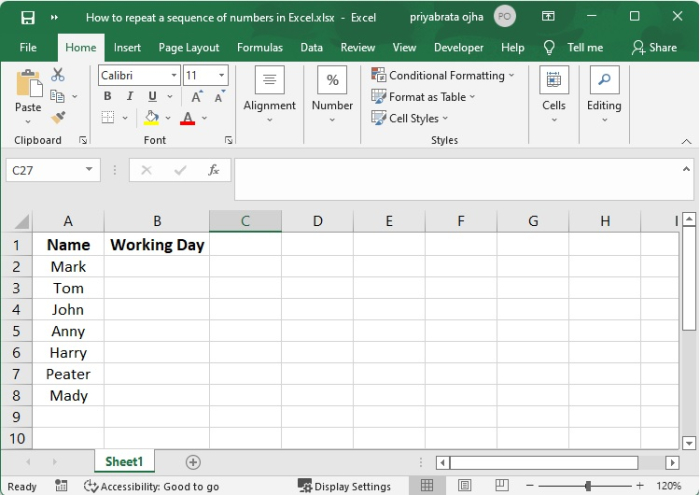Step 2

To begin with, manually enter 1 into field B2 as the number of working days for Mark. After that, go to cell B3 and make your selection.

After that, enter the MOD function that you copied into the Formula Bar. The MOD function is as follows −

=MOD(B2,3)+1


Refer the following image −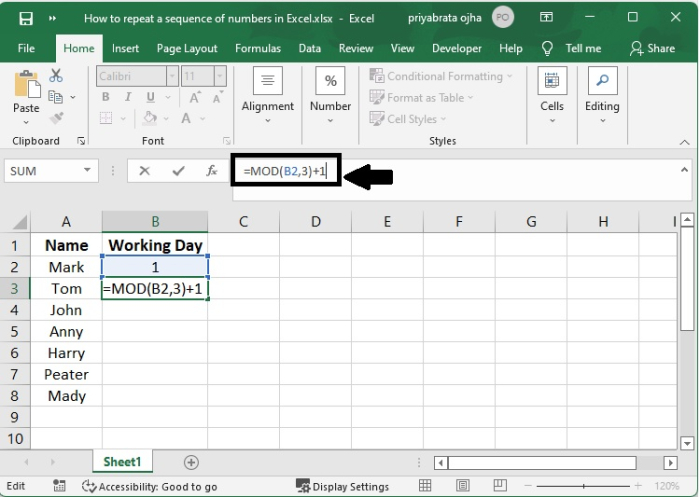The number 3 stands as the dividing number, and +1 represents the rising interval.

Step 3

When you hit the Enter button on your keyboard, you will get the following output −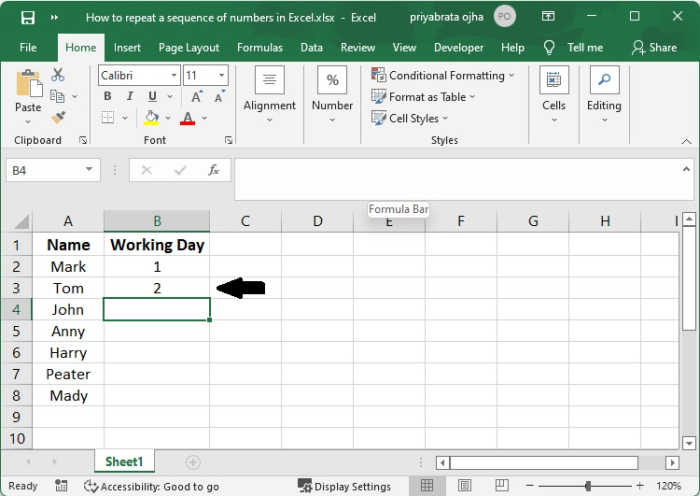Step 4

After that, you need to repeat the number pattern by dragging the autofill indicator lower.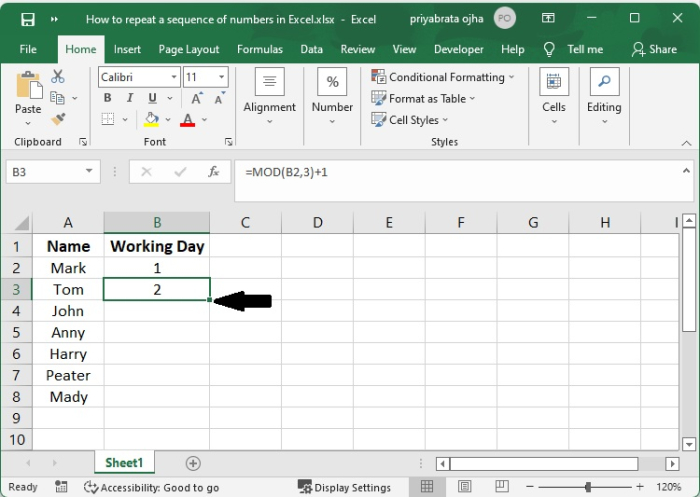Step 5

After that, pull down the autofill until the desired result is shown below.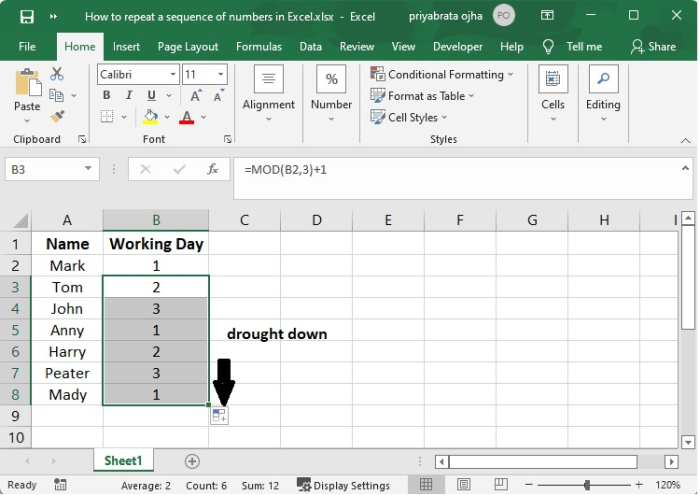Step 6

After you have finished the steps outlined above, you will be presented with a series of repeated numbers. These numbers represent the return value of the MOD function, which is seen in the image below.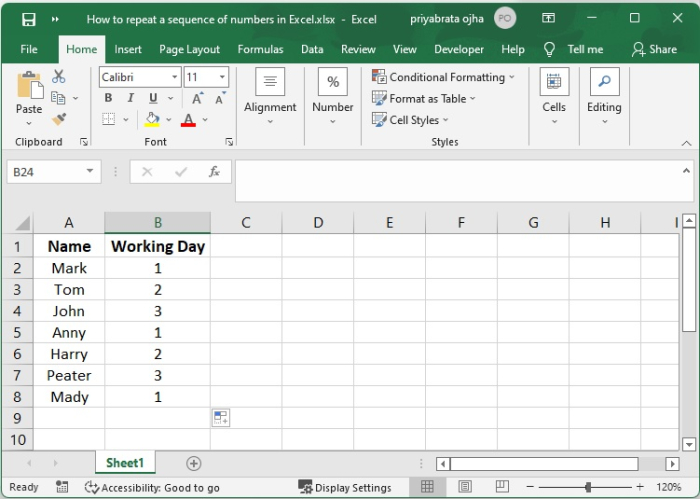## Conclusion

According to the formula shown above, the numbers 1 through 3 are repeated. Changing the number 3 to any other number allows you to make the sequence whatever length you want; this is all that is required if you need to do so.

In order to get an accurate answer from the formula, the sequence number that you use must begin with the number 1. This formula can only be used to repeat a simple number sequence; if you wish to repeat a text string sequence, such as A1001, A1002, and A1003, it will not work for you since this formula only applies to simple number sequences.

Updated on: 10-Sep-2022

10K+ Views